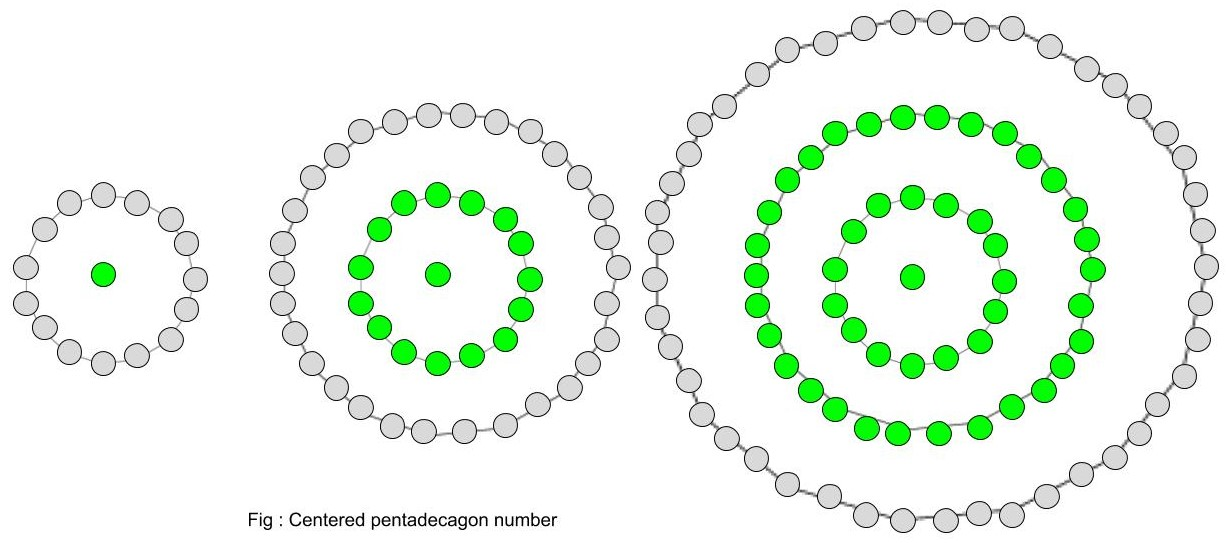Given a number n, find the nth Centered Pentadecagonal Number .

A Centered Pentadecagonal Number represents a dot in the centre and other dots surrounding it in successive pentadecagonal(15-sided polygon) layers.Examples :

```Input :  2
Output : 16

Input : 8
Output : 421
```

## Recommended: Please try your approach on {IDE} first, before moving on to the solution.

n-th term of Centered pentadecagonal number:-

``````

Below is the basic implementation of the above idea.

## C++

 `// C++ Program to find  ` `// nth centered  ` `// pentadecagonal  number ` `#include ` `using` `namespace` `std; ` ` `  `// centered pentadecagonal function ` `int` `center_pentadecagonal_num(``long` `int` `n) ` `{ ` `    ``// Formula to calculate nth ` `    ``// centered pentadecagonal number ` `    ``return` `(15 * n * n - 15 * n + 2) / 2; ` `} ` ` `  `// Driver Code ` `int` `main() ` `{ ` `    ``long` `int` `n = 3; ` `    ``cout << n << ``"th number : "`  `             ``<< center_pentadecagonal_num(n); ` `    ``cout << endl; ` `    ``n = 10; ` `    ``cout << n << ``"th number : "` `             ``<< center_pentadecagonal_num(n); ` ` `  `    ``return` `0; ` `} `

## Java

 `// Java Program to find nth centered  ` `// pentadecagonal number ` ` `  `import` `java.io.*; ` ` `  `class` `GFG { ` `     `  `    ``// centered pentadecagonal function ` `    ``static` `long` `center_pentadecagonal_num(``long` `n) ` `    ``{ ` `     `  `        ``// Formula to calculate nth ` `        ``// centered pentadecagonal number ` `        ``return` `(``15` `* n * n - ``15` `* n + ``2``) / ``2``; ` `    ``} ` `     `  `    ``// Driver Code ` `    ``public` `static` `void` `main (String[] args)  ` `    ``{ ` `     `  `        ``long` `n = ``3``; ` `        ``System.out.print(n + ``"th number : "``); ` `        ``System.out.println(  ` `                  ``center_pentadecagonal_num(n)); ` `         `  `        ``n = ``10``; ` `        ``System.out.print( n + ``"th number : "``); ` `        ``System.out.println( ` `                 ``center_pentadecagonal_num(n)); ` `    ``} ` `} ` ` `  `// This code is contributed by ajit. `

## Python3

 `# Program to find nth ` `#centered pentadecagonal number ` ` `  `def` `center_pentadecagonal_num(n) : ` `     `  `    ``# Formula to calculate nth ` `    ``# centered pentadecagonal number ` `    ``return` `(``15` `*` `n ``*` `n ``-` `15` `*` `n ``+` `2``) ``/``/` `2` ` `  `# Driver Code ` `if` `__name__ ``=``=` `'__main__'` `: ` `         `  `    ``n ``=` `3` `    ``print``(n,``"rd number : "``, ` `                ``center_pentadecagonal_num(n)) ` `    ``n ``=` `10` `    ``print``(n,``"th number : "``, ` `                 ``center_pentadecagonal_num(n)) ` `                  `  ` `  `# This code is contributed  by m_kit `

## C#

 `// C# Program to find  ` `// nth centered  ` `// pentadecagonal number ` `using` `System; ` ` `  `class` `GFG ` `{ ` `     `  `    ``// centered  ` `    ``// pentadecagonal function ` `    ``static` `long` `center_pentadecagonal_num(``long` `n) ` `    ``{ ` `     `  `        ``// Formula to calculate  ` `        ``// nth centered  ` `        ``// pentadecagonal number ` `        ``return` `(15 * n * n - ` `                ``15 * n + 2) / 2; ` `    ``} ` `     `  `    ``// Driver Code ` `    ``static` `public` `void` `Main () ` `    ``{ ` `        ``long` `n = 3; ` `        ``Console.Write(n + ``"th number : "``); ` `        ``Console.WriteLine(  ` `                ``center_pentadecagonal_num(n)); ` `         `  `        ``n = 10; ` `        ``Console.Write( n + ``"th number : "``); ` `        ``Console.WriteLine( ` `                ``center_pentadecagonal_num(n)); ` `    ``} ` `} ` ` `  `// This code is contributed by ajit. `

## PHP

 ` `

Output :

```3th number : 46
10th number : 676
```

Attention reader! Don’t stop learning now. Get hold of all the important DSA concepts with the DSA Self Paced Course at a student-friendly price and become industry ready.

My Personal Notes arrow_drop_upCheck out this Author's contributed articles.

If you like GeeksforGeeks and would like to contribute, you can also write an article using contribute.geeksforgeeks.org or mail your article to contribute@geeksforgeeks.org. See your article appearing on the GeeksforGeeks main page and help other Geeks.

Please Improve this article if you find anything incorrect by clicking on the "Improve Article" button below.

Improved By : jit_t